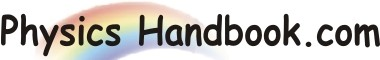HOME TOPICS DEFINITIONS TABLES LAWS INVENTIONS EXPERIMENTS QUIZ VIDEOS
 A B C D E F G H I J K L M N O P Q R S T U V W X Y Z
Electromagnetic Waves
According to Maxwell, a changing magnetic field produce a changing electric field. The changing electric field in turn produces a changing magnetic field and so on. In fact ,the two field act as the sources of each other. Therefore, in order to initiate an electromagnetic wave, we must create a changing electric field .Once we do this, the self regenerating process occurs and the electromagnetic wave propagates of its own.
We create changing fields when we alter the motion of electric charge i.e. we create changing fields when we alter the motion of electric charge i.e. we accelerate the charge.- Accelerated electric charge is the source of electromagnetic waves.
(I) In an LC circuit, the charge oscillate (i.e. undergoing acceleration) across the capacitor plates. Therefore, an Lc circuit emits electromagnetic waves which have the same frequency as that of the oscillating charge.
(II) The emission of visible light is associated with electrons jumping from higher energy orbit to lower energy orbit.
(III) X-rays are produced when fast moving electrons abruptly decelerate as they collide with a metal target of high atomic number.
All electromagnetic waves are similar except for their wavelength and frequency but this difference is very important. For example, visible light is one kind of EM wave having frequency order of 1014Hz.It is not possible to generate light wave using electronic circuits. What about the electromagnetic waves whose frequencies lie above and below the visible range? Such electromagnetic waves are invisible and can be ducted or measured by physical means.